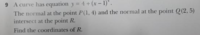# Questions about tangents and normals

#### QueekJJ

##### New memberI had tried many different ways to understand this question, but i cant seem to find what the question really wants from me. I understand the curve equation, its just that the 2 normal points (P and Q) doesnt make sense to me. Could someone maybe explain what am I to do? Thanks#### R.M.

##### Junior Member
A normal at a point is perpendicular to the tangent line at that same point. What is the relationship between the slopes of perpendicular lines?

#### Dr.Peterson

##### Elite Member
View attachment 25874

I had tried many different ways to understand this question, but i cant seem to find what the question really wants from me. I understand the curve equation, its just that the 2 normal points (P and Q) doesnt make sense to me. Could someone maybe explain what am I to do? ThanksYou can find the slope of the curve at each point, right? (Hint: use the derivative)

Then, you were asked if you know how the slopes of perpendicular lines are related. (Hint: negative reciprocal)

Then you can find the slopes of the normal lines, and find their equations, and find their intersection.

Which parts of that are you struggling with?